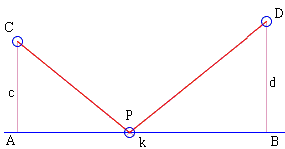# Huygens' Problem

Huygens' problem is superficially similar to that of Heron, but both the methods of solution and the final results are essentially different.

Given a straight line $\lambda$ and points C and D on the same side from $\lambda$. Find on $\lambda$ point $P$ that minimizes $CP^{2}+DP^{2}$.(Just to remind, Heron's problem asked to minimize $CP+DP$.

Solution## Solutions

Given a straight line $\lambda$ and points C and D on the same side from $\lambda$. $A$ and $B$ are projections of points $C$ and $D$ on $\lambda$. Find on $\lambda$ point $P$ that minimizes $CP^{2}+DP^{2}$.The problem admits an algebraic solution. Define $A$ and $B$ as projections of points $C$ and $D$ on $\lambda$. Denote for simplicity $AC=c$, $BD=d$, $AB=k$, and the unknown distance $CP=x$. According to the Pythagorean theorem or the formula for the Euclidean distance, we are to minimize

$CP^{2}+DP^{2} = (c^{2}+x^{2})+(d^2+(k-x)^{2})=(c^{2}+d^{2}+k^{2}) + (2x^2-2kx)$.

Introduce function $f(x)=2x^2-2kx=2x(x-k)$. Since, $c^{2}+d^{2}+k^{2}$ is a constant for a given configuration, the minimum we are after is attained for the $x$ that minimizes $f(x)$.

Function $f(x)$ is a parabola with the roots at $0$ and $k$ so that its axis and the minimum are at $x=k/2$. Thus, unlike in Heron's problem, the answer does not depend on $c$ or $d$.

### References

1. P. J. Nahin, When Least Is Best, Princeton University Press, 2007 (Fifth printing).### A Sample of Optimization Problems III

• Mathematicians Like to Optimize
• Mathematics in Pizzeria
• The Distance to Look Your Best
• Building a Bridge
• Linear Programming
• Residence at an Optimal Distance
• Distance Between Projections
• Optimization in a Crooked Trapezoid
• Greatest Difference in Arithmetic Progression
• Area Optimization in Trapezoid
• Minimum under Two Constraints
• Optimization with Many Variables
• Minimum of a Cyclic Sum with Logarithms
• A Problem with a Magical Solution from Secrets in Inequalities
• Leo Giugiuc's Optimization with Constraint
• Problem 4033 from Crux Mathematicorum
• An Unusual Problem by Leo Giugiuc
• A Cyclic Inequality With Constraint in Two Triples of Variables
• Two Problems by Kunihiko Chikaya
• An Inequality and Its Modifications
• A 2-Variable Optimization From a China Competition
•